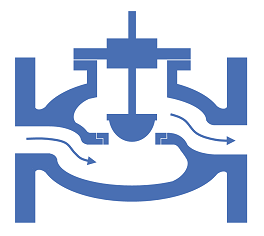f
Neutrium

# Cv

Cv versus Orifice SizeThe pressure drop or flow rate through a valve or orifice plate is typically calculated using the a flow coefficient, Cv or orifice diameter. This article demonstrates how to convert between these two parameters when performing functions such as selecting a valve with an equivalent pressure drop to a given orifice plate.

Converting Between Cv, Kv and KThere are several common ways to express the losses caused by pipe fittings and equipment. Depending on the calculation programs or methods available and engineer may require to convert between one form or another. This article details the equations required to convert between the resistance coefficient and flow coefficient methods (K, Cv and Kv).

Pressure Loss from Fittings in Pipe Summary

Fittings such as elbows, tees, valves and reducers represent a significant component of the pressure loss in most pipe systems. This article discusses the differences between several popular methods for determining the pressure loss through fittings. The methods discussed for fittings are: the equivalent length method, the K method (velocity head method or resistance coefficient method), the two-K method and the three-K method. In this article we also discuss method for calculating pressure loss through pipe size changes as well as control valves.

Pressure Loss Cv and Kv MethodCv and Kv are singles values in units of flowrate that may be used to characterise the relationship between flowrate and pressure loss for fittings and equipment. This article demonstrates how to calculate the Cv or Kv values, and how to use these values to determine the pressure loss for a given flowrate.